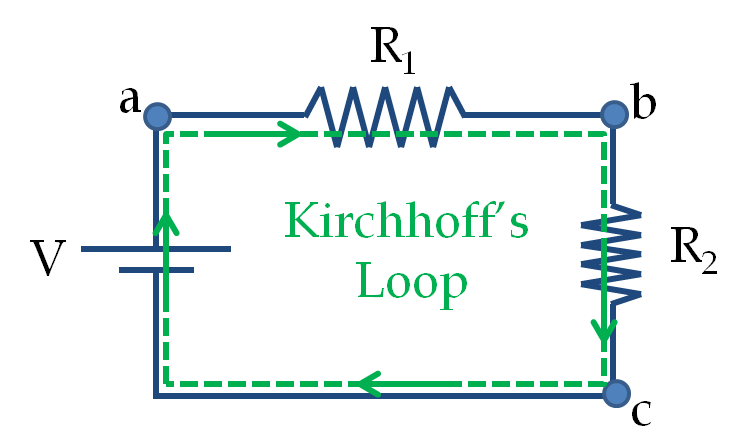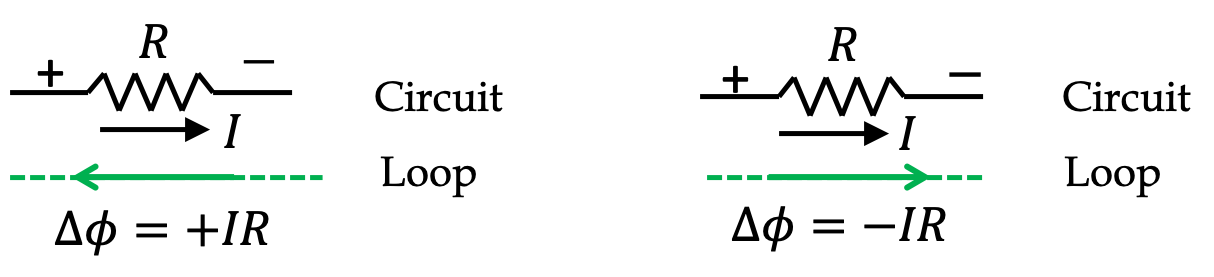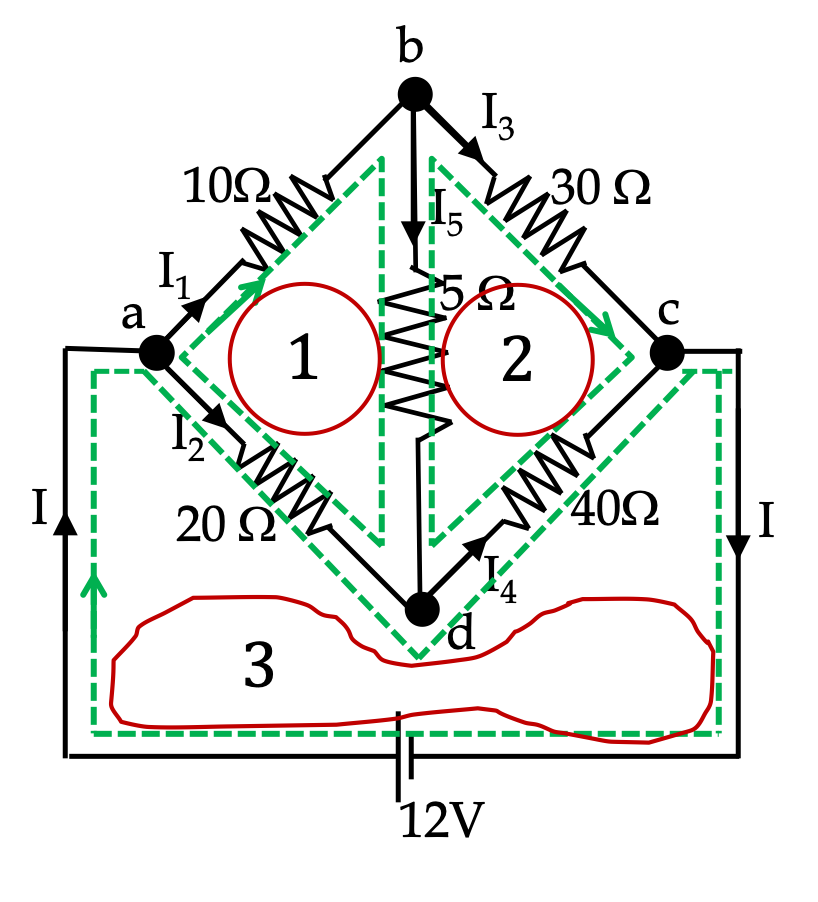## Section36.5Kirchhoff's Rules

Conservation of charge and energy in the circuit provide us with two rules that are called Kirchhoff's current law (KCL) and Kirchhoff's voltage law (KVL). They are also called the node rule and the loop rule respectively.

The Node Rule or Kirchhoff's current law (KCL)

In a circuit, charges do not accumulate or deplete from any point - any charge leaving a point by way of an outgoing current is replenshed by charges arriving by way of incoming current. The rate of leaving and arrival must equal due to the principle of conservaiton of charge.

Suppose we represent current comming to a point by a positive number and that leaving by a negative number, then we will find that sum of incoming and outgoing currents must equal zero (Figure 36.5.1). That is, algebraic sum of all currents must be zero.

\begin{equation} \sum I = 0.\label{eq-KCL}\tag{36.5.1} \end{equation}

When we apply this law to nodes of a circuit, we get relations among currents in various branches of the circuit.

The Loop Rule or Kirchhoff's voltage law (KVL)

Suppose we start at some point in an electric circuit and move some charge $q$ across various circuit elements, finally arriving at the initial place, thus making a closed loop. Since final place is same as the initial place, net change in energy of the charge will be zero.

\begin{equation*} \sum_\text{steps across elements} U = 0. \end{equation*}

Since potentials across circuit elements are usually different, when you go across an element, the energy of the charge goes up or goes down by $q\Delta\phi\text{,}$ goes up if $\Delta\phi \gt 0$ and goes down if $\Delta\phi \lt 0\text{.}$ These increases and decreases must sum to zero as illustrated in Figure 36.5.2.Figure 36.5.2. Kirchhoff's voltage law or the loop rule: $\sum_\text{around a loop} \Delta\phi= 0\text{.}$

Since $q$ is a common factor in this sum, we can just sum potential differences in each step and the sum should equal zero.

\begin{equation} \sum_\text{around a loop} \Delta\phi= 0.\tag{36.5.2} \end{equation}

This is the loop rule or Kirchhooff's voltage law (KVL). Applying this law to loops in circuits gives us relations among the potential values $\phi_i$ at various nodes of the circuit. These potential values are sometimes also called voltage values at those points - these values are potential difference with respect to a point chosen to be zero of the potential, which is usually the negative of some power source.

### Subsection36.5.1Sign Conventions for Voltage in Loop Equation

To apply the Kirchhoff's Loop Rule, it is often helpful to follow a sign convention for currents and voltages for consistency in algebraic manipulations. While electric circuit is a fixed structure, Kirchhoff's loop is arbitrary loop. Hence, the direction of voltages and currents in the actual circuit may differ from the way you traverse a particular Kirchhoff's loop. Therefore, it is important that you maintain a sign convention that assigns increase or decrease in voltage consistently across all your calculations.

Sign Convention for Voltage Sources

For potentials across a voltage source, we note that potential drops from a higher value to a lower value when you go from the positive terminal of a battery to its negative terminal, but if you go in the other direction, the potential will increase across the battery. Therefore, when we go from the negative to the positive of a voltage source, the change in potential is positive and when we go from positive to the negative of the source the change in potential is negative as shown in Figure 36.5.3.

Sign Convention for Voltages Across Resitors

Similarly, when we assume a direction of current in a resistor, we are actually assuming one side of the resistor as being at a higher potential than the other side since the current flows from the higher potential towards the lower potential.

In a circuit we may not know the direction of a current a-priori, but we must pick a direction in order to proceed in calculations. Therefore, we label the two ends of a resistor with $+$ and $-$ to indicate the sides presumed to be at higher and lower voltages respectively. Although such labeling is not necessary but it often helps avoid silly mistake. This is illustrated in Figure 36.5.4.Figure 36.5.4. Using sign convention shows that voltage drop across can be $+IR$ or $-IR$ depending upon the direction of traversal in the Kirchhoff's voltage loop.

Zero Voltage Reference in a Circuit with Multiple Voltage Sources

When you have multiple voltage sources in a circuit, you can choose the negative end of only one of the sources. The negative ends of only those sources which are directly connected to the zero potential chosen will be at zero potential. The voltage of the source tells us by how much potential changes when you go across from the negative end to the positive end.

### Subsection36.5.2Applying KCL and KVL

In a circuit with given resitors and voltage sources, there are two types of unknowns:

1. Currents through each circuit element, i.e., through each resistor and through each voltage source, or alternately, in each branch of the circuit.
2. Electric potential drop across each circuit element, i.e., across each resistor and across each voltage source.

From potential drops across every resistor we can us Ohm's law to find current through them, and using KCL then lets us find current through the voltage sources. On the other hand, from current through each resistor, we can us Ohm's law to find potential drops across each resistor.

Therefore, we can choose to set up algebraic quations for unknown currents or unknown potentials. Since current through all elements that are connected in series would be same, i.e., there is one current per branch rather than one current for each element, it is often easier to set up equations for currents. But if you are careful with potential drops, same amount of algebra is involved in that approach. In the end, it is up to you to see which way to proceed.

Find current through each resistor in the circuit in Figure 36.5.6. The values are: $R_1 = 10\ \Omega\text{,}$ $R_2 = 20\ \Omega\text{,}$ $R_1 = 30\ \Omega\text{,}$ $V_1 = 10\ \text{V}\text{,}$ $V_2 = 15\ \text{V}\text{.}$

Hint

1. Pick specific directions for the three currents. 2. One KCL equation. 3. Two KVL equations, wher make sure to use the correct signs.

$I_1=\dfrac{1}{22}\, \text{ A},\ I_2=\dfrac{6}{22}\, \text{ A},\ I_3=\dfrac{7}{22}\, \text{ A}.$

Solution

First we pick currents in each branch and label the resistors with $+$ and $-$ across each resistor based on our choice of direction of current as shown in Figure 36.5.7. Note that, at this stage I do not know the directions of the currents, but I must pick these directions based on intuition or arbitrarily.

The directions are then used to assign $+$ and $-$ across each resistor. These will be used to assign pick up voltage or drop off voltage in the Kirkchoof's loop equations. We also recognize nodes where potential values are unique. There are four such nodes, labeled, $a\text{,}$ $b\text{,}$ $c\text{,}$ and $d\text{.}$

There are two junctions in the diagram where currents meets. We get the same KCL equation from them.

\begin{equation} I_1 + I_2 - I_3 = 0.\label{eq-exp-kirk-two-source-three-loop-KCL}\tag{36.5.3} \end{equation}

This gives us one equation in three unknowns. Therefore, we need to generate two other equations, which will come from KVL applied to two loops in the circuit.

Let's apply KVL in loop $a-b-c-a\text{.}$

In the $a-b$ step, we are going in the same direction in the math loop as the current in the resistor. Therefore, the potential will drop by $I_3R_3\text{,}$ which is evident in going from $+\text{ to }-$ of the element. Then, in step $b-c\text{,}$ we go from $-\text{ to }+\text{,}$ which will mean increase of potential by $V_1\text{.}$

Finally, in step $c-a\text{,}$ we go from $+\text{ to }-\text{,}$ therefore, there will be a drop of potential of magnitude $I_1R_1\text{.}$ We get

\begin{equation*} -I_3R_3 + V_1 - I_1R_1 = 0. \end{equation*}

Using the numerical values we get

\begin{equation} -30 I_3 + 10 - 10 I_1 = 0.\label{eq-exp-kirk-two-source-three-loop-KVL-1}\tag{36.5.4} \end{equation}

Finally, we apply KVL to the outer loop $a-d-b-c-a\text{.}$

In step $a-d$ we will have increase of $I_2R_2$ since, in the loop, we are going against the direction of the current in the resistor, or equivalently, we are going from $-\text{ to }+$ of the assigned signs to the resistor. In step $a-d\text{,}$ the potential drops by $V_2\text{.}$ In step $b-c\text{,}$ potential goes up by $V_1$ and in step $c-a\text{,}$ potential drops by $I_1R_1\text{.}$

Therefore,

\begin{equation*} +I_2R_2 -V_2 + V_1 - I_1R_1 = 0. \end{equation*}

Using the numerical values we get

\begin{equation} +20 I_2 -15 + 10 - 10 I_1 = 0.\label{eq-exp-kirk-two-source-three-loop-KVL-2}\tag{36.5.5} \end{equation}

You can solve Eqs. (36.5.3), (36.5.4), and (36.5.5) by method of elimination or some other method you are more familiar with. It is very common to make mistakes in these calculations, so, you should pluc back your answer into these equations to check your answer. I used the command “Solve x+y=z, 10x+30z=10, 10x -20y=-5” at Wolfram Alpha website. My answers are

\begin{equation*} I_1=\dfrac{1}{22}\, \text{ A},\ I_2=\dfrac{6}{22}\, \text{ A},\ I_3=\dfrac{7}{22}\, \text{ A}. \end{equation*}

Since I got all of these current magnitude positive, my original directions were correct. If I had got any of them negative, it would tell me that the direction of the current I picked in the figure above should have been the oposite direction.

In a bridge circuit, resistors and other elements in a circuit form a bridge-like structure. Bridge circuits are used for many purposes in engineering and physics. Find currents in each wire of the bridge circuit given in Figure 36.5.11.

Hint

Systematically set up KCL and KVL equations.

$I = \dfrac{78}{155}\, \text{ A}\text{,}$ $I_1 = \dfrac{51}{155}\, \text{ A}\text{,}$ $I_2 = \dfrac{27}{155}\, \text{ A},$ $I_3 = \dfrac{45}{155}\, \text{ A}\text{,}$ $I_4 = \dfrac{33}{155}\, \text{ A}\text{,}$ $I_5 = \dfrac{6}{155}\, \text{ A}.$

Solution

Using current conservations at nodes we get three equations from four nodes, the KCL on the fourth node is just a sum of the other relations.

\begin{align} \amp \text{node a: } I = I_1 + I_2 \label{exp-kirk-bridge-circuit-KCL-1}\tag{36.5.6}\\ \amp \text{node b: } I_1 = I_3 + I_5\label{exp-kirk-bridge-circuit-KCL-2}\tag{36.5.7}\\ \amp \text{node d: } I_2 + I_5 = I_4\label{exp-kirk-bridge-circuit-KCL-3}\tag{36.5.8} \end{align}

These give us three relations among six unknowns, $I,\ I_1,\ I_2,\ I_3,\ I_4,\ I_5\text{.}$ Therefore, we need three loops equations from KVL. We can pick any three loop in the circuit. My choice is shown in figure below.The KVL equations for the three loops are

\begin{align} \amp -10 I_1 - 5 I_5 + 20 I_2 = 0 \label{exp-kirk-bridge-circuit-KVL-1}\tag{36.5.9}\\ \amp -30 I_3 + 40 I_4 + 5 I_5 = 0\label{exp-kirk-bridge-circuit-KVL-2}\tag{36.5.10}\\ \amp -20 I_2 -40 I_4 + 12 = 0\label{exp-kirk-bridge-circuit-KVL-3}\tag{36.5.11} \end{align}
Solving Eqs. (36.5.6) to (36.5.11) simultaneously will give the values of the six currents in the circuit. You can systematically apply the method of elimination. Very tedious to do that. I will carefully type command at Wolfram Alpha website. Here is the command I used, “Solve a = a1 + a2, a1 = a3 + a5, a2 + a5 = a4, -10 a1 - 5 a5 + 20 a2 = 0, -30 a3 + 40 a4 + 5 a5 = 0, -20 a2 - 40 a4 + 12 = 0,” which gave me the following answer.
\begin{align*} \amp I = \dfrac{78}{155}\, \text{ A}, I_1 = \dfrac{51}{155}\, \text{ A}, I_2 = \dfrac{27}{155}\, \text{ A},\\ \amp I_3 = \dfrac{45}{155}\, \text{ A}, I_4 = \dfrac{33}{155}\, \text{ A}, I_5 = \dfrac{6}{155}\, \text{ A}. \end{align*}

In a bridge circuit, resistors and other elements in a circuit form a bridge-like structure. You can analyze the circuit given in Figure 36.5.13 by setting up six equations for six currents. That is not the most economical way for analyzing this circuit since it has only four unique values of electic potentials at four nodes in the circuit. The values of potentials at two of the nodes is already known. Therefore, we need to find only two unknown potentials. Find values of electric potential at the four nodes in the circuit.

Hint

Express each current in terms potentials and then use them in current conservation law, KCL.

$\phi_a = 12\text{ V}\text{,}$ $\phi_b=0\text{,}$ $\phi_c = \dfrac{270}{31}\text{ V}\text{,}$ $\phi_d = \dfrac{264}{31}\text{ V}\text{.}$

Solution

Let $\phi_a\text{,}$ $\phi_b\text{,}$ $\phi_c\text{,}$ and $\phi_d$ denote electric potentials at $a,\ b,\ c,\ d$ respectively. Since voltage source maintains potential difference across it,

\begin{equation*} \phi_a - \phi_b = 12\text{ V}. \end{equation*}

We can always, choose one place to be zero reference of electric potential. As customary, we will choose it to be the negative of a voltage source. Note, if we had multiple voltage sources, negative of only one of them can be set to zero.

\begin{equation*} \phi_b = 0,\ \phi_a= 12\text{ V}. \end{equation*}

From the circuit we have the following for Ohm's law for each resistor.

\begin{align*} \amp 12- \phi_c = 10 I_1\\ \amp 12- \phi_d = 20 I_2\\ \amp \phi_c -0 = 30 I_3\\ \amp \phi_d -0 = 40 I_4\\ \amp \phi_c - \phi_d = 5 I_5 \end{align*}

We will use these to replace currents in the following KCL equations at nodes $c$ and $d\text{,}$

\begin{align*} \amp I_1 = I_3 + I_5,\\ \amp I_4 = I_2 + I_5, \end{align*}

which gives

\begin{align*} \amp \dfrac{12-\phi_c}{10} = \dfrac{\phi_c}{30} + \dfrac{\phi_c - \phi_d}{5},\\ \amp \dfrac{ \phi_d}{40} = \dfrac{12-\phi_d}{20} + \dfrac{\phi_c - \phi_d}{5}. \end{align*}

These can be simplified to

\begin{align*} \amp 10 \phi_c - 6 \phi_d = 36,\\ \amp -8 \phi_c + 11 \phi_d = 24. \end{align*}

Solving them yields

\begin{equation*} \phi_c = \dfrac{270}{31}\text{ V},\ \ \phi_d = \dfrac{264}{31}\text{ V}. \end{equation*}

From the potentials, it is trivial to get the currents now.